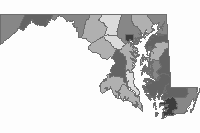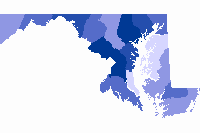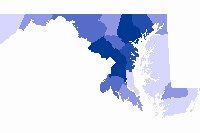Description

more
The percentage of a school’s food budget that is spent on locally grown or processed food products during the 2011-2012 academic school year. Please note that the definition of local varies across school districts. Data source: United States Department of Agriculture, Farm to School Census Date: 2013

Dataset Attributes

• OBJECTID
Number
• Shape
Number
• NAME
Text
{"value"=>"Worcester", "count"=>1} (), {"value"=>"Carroll", "count"=>1} (), {"value"=>"Dorchester", "count"=>1} (), {"value"=>"Talbot", "count"=>1} (), {"value"=>"Cecil", "count"=>1} (), {"value"=>"Charles", "count"=>1} (), {"value"=>"Caroline", "count"=>1} (), {"value"=>"Calvert", "count"=>1} (), {"value"=>"Anne Arundel", "count"=>1} (), {"value"=>"Allegany", "count"=>1} ()
• Percent_Lo
Text
{"value"=>"1% - 2%", "count"=>6} (), {"value"=>"Data Not Available", "count"=>4} (), {"value"=>"16% - 20%", "count"=>3} (), {"value"=>"0%", "count"=>2} (), {"value"=>"21% - 25%", "count"=>2} (), {"value"=>"3% - 5%", "count"=>2} (), {"value"=>"6% - 10%", "count"=>2} (), {"value"=>"26% - 30%", "count"=>2} (), {"value"=>"11% - 15%", "count"=>1} ()
• Shape_Length
Number
• Shape_Area
Number
• Estimate
Number
{"value"=>1.5, "count"=>6, "code"=>1.5} (), {"value"=>"Data Withheld", "count"=>4, "code"=>-99999} (), {"value"=>23, "count"=>2, "code"=>23} (), {"value"=>4, "count"=>2, "code"=>4} (), {"value"=>8, "count"=>2, "code"=>8} (), {"value"=>18, "count"=>2, "code"=>18} (), {"value"=>28, "count"=>2, "code"=>28} (), {"value"=>17, "count"=>1, "code"=>17} (), {"value"=>13, "count"=>1, "code"=>13} ()

• By on September 30, 2016
• Updated 2 months ago

Related Datasets

• Percent Free and Reduced MealsPercent Free and Reduced Meals
• Dollars Spent on FoodDollars Spent on Food
• Total Number of BreakfastsTotal Number of Breakfasts
• Total Number of LunchesTotal Number of Lunches